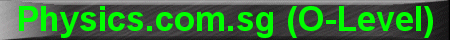(New tips are continually added to these pages.  Check back in a few months' time for more)

TOPIC 3:     Forces and Moments

Tip 1:

Every student knows F = ma.   But what's F?

F is the Resultant Force, or Net Force.

For example, if I push a 5 kg box with a force of 30 N to the right, and you push the same box with a force of 20 N to the left, then the Resultant Force is 10 N to the right (I win).

The box will then accelerate towards the right at 2 m s-2, assuming that friction and air resistance are negligible.

Tip 2:

The moment of a force about a pivot is defined as the product of the force F and the perpendicular distance d from the line of action of the force to the pivot.

Many students just remember Moment = Force x Distance, when in fact the most important key words are perpendicular distance and line of action.Since "O" level problems are relatively simple, most of the time you will get the right answer just by using the formula Moment = Force x Distance, but do keep the above diagram in mind, especially when you proceed to the "A" levels.